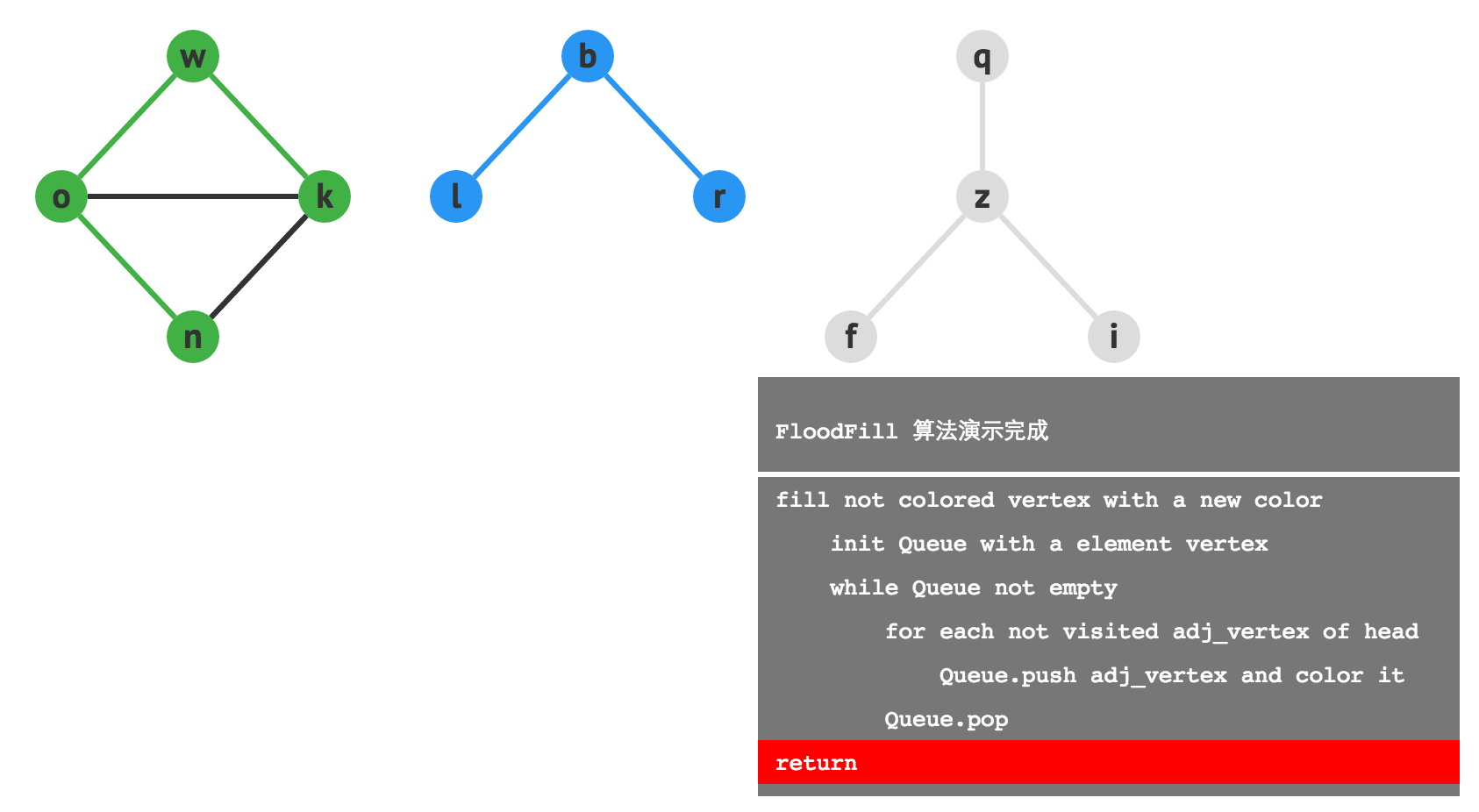# 【数据结构与算法学习笔记十四】floodfill算法

FloodFill算法通常译作“洪水灌溉法”，算法通过给图中的顶点染色，最终使得同一个连通分量的顶点颜色相同，不同连通分量的顶点颜色不同。算法的描述如下：

1. 找到一个没有染色的顶点，将其染为新的颜色 Color_new，如果没有则算法结束。
2. 初始化一个空的队列，并将第一步的顶点插入队列。
3. 不断获得队首元素的值并弹出，将和队首元素相邻的未染色顶点染为Color_new，并将其加入队列。

4. 重复执行第一步，直到所有顶点都被染色，算法结束。FloodFill 的时间复杂度是 O(V+E)，其中广度优先遍历的部分可以替换成深度优先遍历，复杂度是一样的。通常考虑到递归调用的时间开销，往往广度优先遍历的效率要更高一些。

#include <iostream>
#include <vector>
#include <cstring>
#include <queue>

using namespace std;

class Graph {
private:
int n;
int * color;
vector<int> * edges;

public:
Graph(int input_n) {
n = input_n;
edges = new vector<int>[n];
color = new int[n];
memset(color, 0, n * sizeof(int));
}

~Graph() {
delete[] edges;
delete[] color;
}

void insert(int x, int y) {
edges[x].push_back(y);
edges[y].push_back(x);
}

void floodfill() {
//首先定义变量color_cnt表示当前染色的值
int color_cnt = 0;
//接下来，枚举所有未染色的顶点。顶点的染色结果记录在
//color数组中，未染色时结果为0，否则为一个正数。因为我们判断一个顶点是否
//染色时，只需要判断color数组中对应的值是否为0即可。
for (int i=0; i<n; i++) {
if (color[i] == 0) {
//接下来，要从当前顶点出发，对所在连通分量进行广度优先遍历。
//每次对所在连通分量的遍历，都会讲连通分量内的所有顶点染为一种
//新的颜色。我们只需要将这些颜色区分开就可以，这里我们不妨让每
//个连通分量染色的值和上次相比都加1.比如没一个连通分量染色的值
//为1.第二个染色的值为2，依次类推。
//在处理每一个连通分量时，第一步要做的就是将color_cnt的值加1，
//并将第i个顶点染为color_cnt这个颜色。
color_cnt++;
color[i] = color_cnt;
//进行广度优先遍历
queue<int> q;
q.push(i);
while(!q.empty()) {
int vertex = q.front();
//枚举和vertex相邻的所有顶点
for(int j: edges[vertex]) {
if(color[j] == 0) {
color[j] = color_cnt;
q.push(j);
}
}
q.pop();
}
}
}
//将color数组结果输出
for(int i=0; i<n; ++i) {
cout << i << ” ” << color[i] << endl;
}
}
};

int main() {
int n, m, k;
cin >> n >> m;
Graph g(n);
for (int i = 0; i < m; ++i) {
int x, y;
cin >> x >> y;
g.insert(x, y);
}
g.floodfill();
return 0;
}